Home Practice
For learners and parents For teachers and schools
Textbooks
Full catalogue
Pricing SupportLog in

We think you are located in United States. Is this correct?

# 2.2 Functions and relations

## 2.2 Functions and relations (EMCF7)

Relation

A rule which associates each element of set $$(A)$$ with at least one element in set $$(B)$$.

Function

A rule which uniquely associates elements of one set $$(A)$$ with the elements of another set $$(B)$$; each element in set $$(A)$$ maps to only one element in set $$(B)$$.

Functions can be one-to-one relations or many-to-one relations. A many-to-one relation associates two or more values of the independent (input) variable with a single value of the dependent (output) variable. The domain is the set of values to which the rule is applied $$(A)$$ and the range is the set of values (also called the images or function values) determined by the rule.

Example of a one-to-one function: $$y = x + 1$$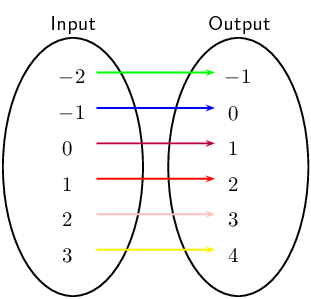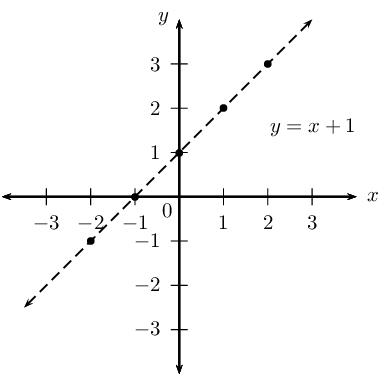Example of a many-to-one function: $$y = x^{2}$$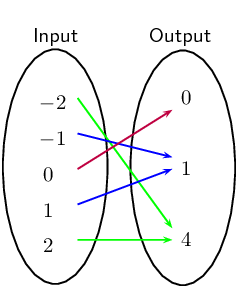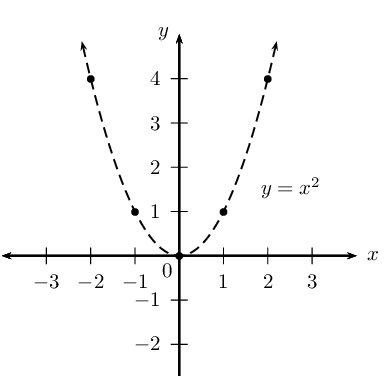However, some very common mathematical constructions are not functions. For example, consider the relation $${x}^{2}+{y}^{2}=4$$. This relation describes a circle of radius $$\text{2}$$ centred at the origin. If we let $$x=0$$, we see that $${y}^{2}=4$$ and thus either $$y=2$$ or $$y=-2$$. This is a many-to-many relation because a single $$x$$-value relates to two different $$y$$-values. Therefore $${x}^{2}+{y}^{2}=4$$ is not a function.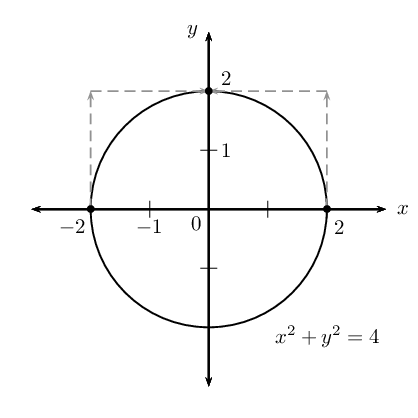Vertical line test

Given the graph of a relation, there is a simple test for whether or not the relation is a function. This test is called the vertical line test. If it is possible to draw any vertical line (a line of constant $$x$$) which crosses the graph of the relation more than once, then the relation is not a function. If more than one intersection point exists, then the intersections correspond to multiple values of $$y$$ for a single value of $$x$$ (one-to-many).

If any vertical line cuts the graph only once, then the relation is a function (one-to-one or many-to-one).

The red vertical line cuts the circle twice and therefore the circle is not a function.

The red vertical line only cuts the parabola once and therefore the parabola is a function.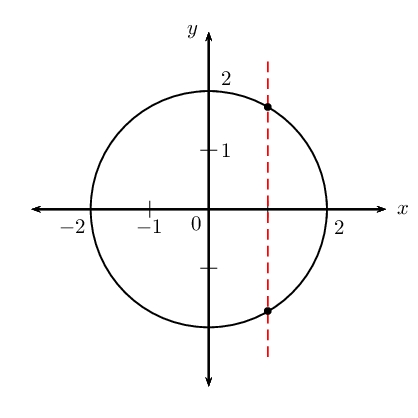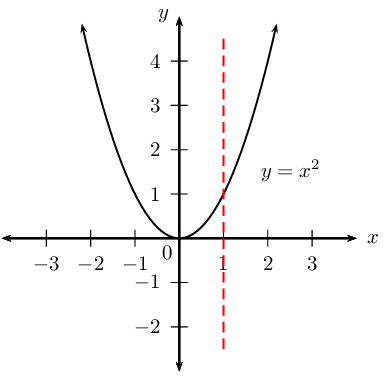## Identifying functions

Textbook Exercise 2.2

Consider the graphs given below and determine whether or not they are functions: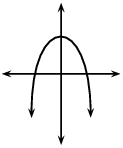Yes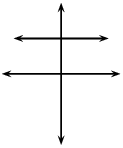Yes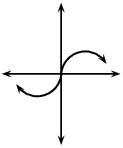Yes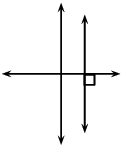No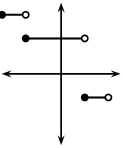Yes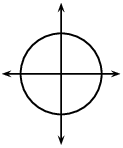No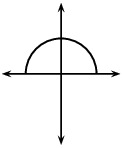Yes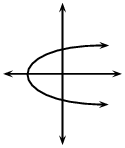No

Sketch the following and determine whether or not they are functions:

$$x + y = 4$$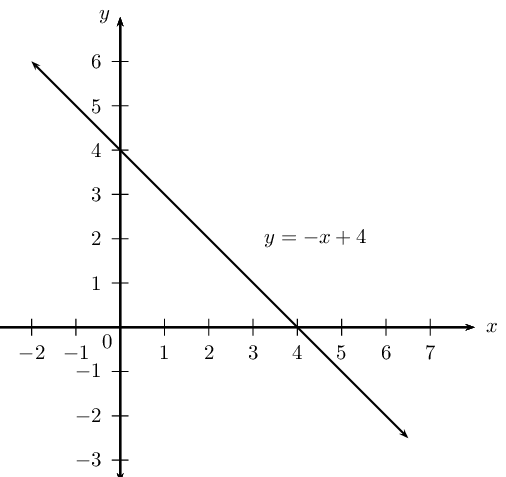$$y = \frac{x}{4}$$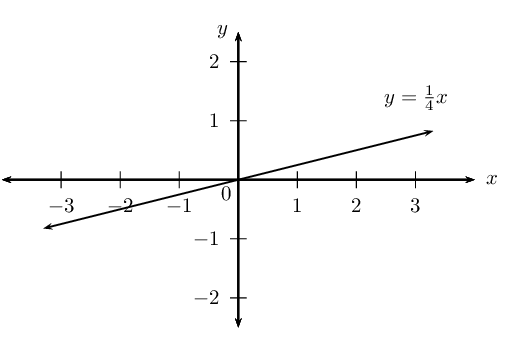$$y = 2^{x}$$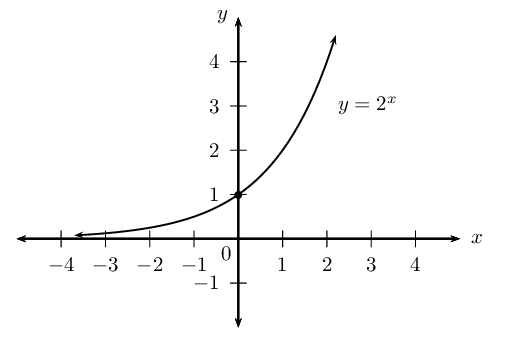$$x^{2} + y^{2} = 9$$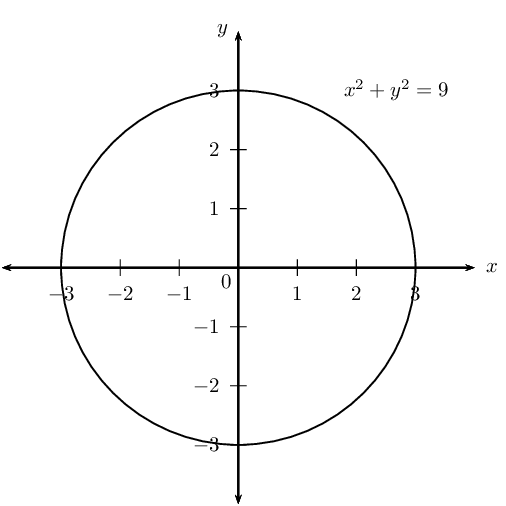$$y = \tan{x}$$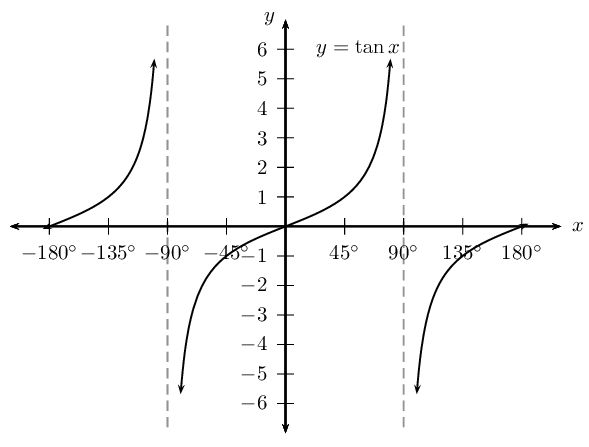The table below gives the average per capita income, $$d$$, in a region of the country as a function of $$u$$, the percentage of unemployed people. Write down an equation to show that the average income is a function of the percentage of unemployed people.

 $$u$$ 1 2 3 4 $$d$$ $$\text{22 500}$$ $$\text{22 000}$$ $$\text{21 500}$$ $$\text{21 000}$$

Per capita income is a measure of the average amount of money earned per person in a certain area.

We see that there is a constant difference of $$-\text{500}$$ between the consecutive values of $$d$$, therefore the relation is a linear function of the form $$y = mx + c$$:

$$u$$ is the independent variable and $$d$$ is the dependent variable.

\begin{align*} d & = mu + c \\ m & = -\text{500} \\ d & = -\text{500}u + c \end{align*}

Substitute any of the given set of values to solve for $$c$$:

\begin{align*} \text{22 500} & = -\text{500}(\text{1}) + c \\ \therefore c & = \text{23 000} \end{align*}

The function is: $$d = -\text{500}u + \text{23 000}$$

Function notation

For the function $$y=f\left(x\right)$$, $$y$$ is the dependent variable, because the value of $$y$$ (output) depends on the value of $$x$$ (input). We say $$x$$ is the independent variable, since we can choose $$x$$ to be any number. Similarly, if $$g\left(t\right)=2t+1$$, then $$t$$ is the independent variable and $$g$$ is the function name.

• If $$h\left(x\right)=3x-5$$ and we need to determine when $$h\left(x\right)=3$$, then we solve for the value of $$x$$ such that:

\begin{align*} h\left(x\right)& = 3x-5 \\ 3 & = 3x - 5 \\ 8 & = 3x \\ \therefore x & = \frac{8}{3} \end{align*}
• If $$h\left(x\right)=3x-5$$ and we need to determine $$h\left(3\right)$$, then we calculate the value for $$h\left(x\right)$$ when $$x=3$$:

\begin{align*} h\left(x\right)& = 3x-5 \\ h\left(3\right)& = 3\left(3\right)-5 \\ & = 4 \end{align*}